# Hands-On-Physics

### GLOSSARYAcceleration is the rate at which the velocity vector changes

### British Thermal Unit (BTU)

A BTU is the amount of energy required to increase the temperature of one pound of water by one degree Fahrenheit. This is equivalent to 1055 Joules. In the United States, the ability of furnaces to warm the air by adding energy and the ability of air conditioners to extract energy from air is expressed in BTUs.

### Calorie

A calorie is defined as the amount of energy required to increase the temperature of a gram water by one degree Celsius. This is equivalent to 4.184 Joules. If you count "calories" when you eat, you are actually counting kilocalories. That is, the "calorie" used in measuring food energy is actually one thousand of the calories we just defined.

### Celsius

In the SI system, temperature is measured in degrees Celsius (C). This scale is defined by: The freezing point of water = 0 C The boiling point of water = 100 C. The boiling point of water changes somewhat with altitude, so it is important to add that 100 C is the boiling temperature of water at sea level. Actually, it is the atmospheric pressure that influences the boiling temperature, so, for accuracy, an average atmospheric pressure at sea level is used, taken as a pressure of 760 mm of mercury.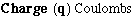Charges are the basis of electricity. A charge exert forces on other charges. The smallest charge is the charge of an electron, 0.000000000000000016 Coulombs.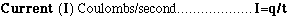Current is the rate of flow of electrical charge.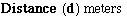One of the undefined qualities of physics, it measures the separation of two points.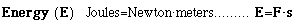The energy of an object increases when work is done on it. An increase in energy may heat up the object, speed it up, lift it up, or all of the above.

### Fahrenheit

The old Fahrenheit scale is still used in the U.S. That temperature scale is defined by: The freezing point of water = 32 F The boiling point of water = 212 F. There are a total of (212 ­p; 32) = 180 Fahrenheit degrees between freezing and boiling, almost twice as many as in the Celsius scale. That means every Celsius degree represents almost twice as large a step as a Fahrenheit degree.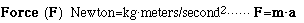The strength of a force is defined by the rate at which it can speed up one kilogram of mass.

A | B | C | D | E | F | G | H | I | J | K | L | M | N
O | P | Q | R | S | T | U | V | W | X | Y | Z
Top of Glossary